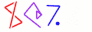Next You can't get there from here 30

# Ouch

• Here's source code for a function I'll call ouch:

```     my \$ouchcode = "sub {
use Acme::HaltingProblem;
my \$arg = shift;
if (halts(\$arg, \$arg)) { 1 while 1 }
else                   { return }
}";
```
• It is expecting to get some program source code as its argument

• Then it asks if that program will halt when given its own source code as input

• Now let's do

```        my \$ouch = eval \$ouchcode;
\$ouch->(\$ouchcode);
```
• We're giving the ouch function its own source code as input

• What does it do?

 NextCopyright © 2005 M. J. Dominus